#Basic Dash Callbacks

This is the 3rd chapter of the Dash Tutorial.
The previous chapter covered the Dash app `layout`
and the next chapter covers interactive graphing.
Just getting started? Make sure to install the necessary dependencies.

In the previous chapter we learned that `set_layout()` describes what the app looks like and is a hierarchical tree of components.
The Dash package provides functions for all of the HTML tags, and the keyword arguments describe the HTML attributes like `style`, `className`, and `id`.
The `dashCoreComponents` package generates higher-level components like controls and graphs.

This chapter describes how to make your Dash apps using callback functions: functions that are automatically called by Dash whenever an input component’s property changes, in order to update some property in another component (the output).

Dash apps should consider the Job Queue,
and horizontal scaling capabilities of Dash Enterprise.

Let’s get started with a simple example of an interactive Dash app.

#### Simple Interactive Dash App

If you’re using Dash Enterprise’s Data Science Workspaces,
copy & paste the below code into your Workspace (see video).

Find out if your company is using Dash Enterprise

``````library(dash)
library(dashCoreComponents)

app <- dash_app()

app %>% set_layout(
html\$h6("Change the value in the text box to see callbacks in action!"),
div(
"Input: ",
dccInput(id = 'my-input', value = 'initial value', type = 'text')
),
br(),
div(id = 'my-output')
)

output(id = 'my-output', property = 'children'),
input(id = 'my-input', property = 'value'),
function(input_value) {
sprintf("Output: \"%s\"", input_value)
}
)

app %>% run_app()
``````
###### Change the value in the text box to see callbacks in action!
Input:

Let’s break down this example:

1. The “inputs” and “outputs” of our application are described
as the arguments of the `add_callback()` function. The first parameter is the output, the second parameter is the input.

2. In Dash, the inputs and outputs of our application are simply the
properties of a particular component. In this example,
our input is the “`value`” property of the component that has the ID
`my-input`”. Our output is the “`children`” property of the
component with the ID “`my-output`”. You can think of the “`children`” property of a component as the content inside it on the webpage.

3. Whenever an input property changes, the function defined as the callback will get called automatically.
Dash provides this callback function with the new value of the input property as
its argument, and Dash updates the property of the output component
with whatever was returned by the function.
4. This callback essentially means that we’re telling Dash to call the callback function whenever the value of the “input” component (the text box) changes in order to update the content (“children”) of the “output” component on the page (the HTML div)..
5. Don’t confuse the `input()` used in `add_callback()` with `dccInput()`. The former is used to describe the inputs of a callback, while the latter is a Dash component.
6. Notice how we don’t set a value for the `children` property of the
`my-output` component in the `layout`. When the Dash app starts, it
automatically calls all of the callbacks with the initial values of the
input components in order to populate the initial state of the output
components. In this example, if you specified the div component as
`div(id = 'my-output', 'Hello world')`,
it would get overwritten when the app starts.
7. The `id` used for the `input()` and `output()` in the callback must match the ID of Dash components on the page.

It’s sort of like programming with Microsoft Excel:
whenever a cell changes (the input), all the cells that depend on that cell (the outputs)
will get updated automatically. This is called “Reactive Programming” because the outputs react to changes in the inputs automatically.

Remember how every component is described entirely through its
arguments? Those arguments that we set in
R become properties of the component,
and these properties are important now.
With Dash’s interactivity, we can dynamically update any of those properties
using callbacks. Often we’ll update the `children` property of HTML
components to display new text (remember that `children` is responsible for the contents of a component) or the `figure` property of a `dccGraph`
component to display new data. We could also update the `style` of a
component or even the available `options` of a `dccDropdown` component!

Let’s take a look at another example where a `dccSlider` updates
a `dccGraph`.

#### Dash App Layout With Figure and Slider

``````library(dash)
library(dashCoreComponents)

app <- dash_app()

file = "https://raw.githubusercontent.com/plotly/datasets/master/gapminderDataFiveYear.csv",
stringsAsFactor = FALSE,
check.names = FALSE
)

continents <- unique(df\$continent)
years <- unique(df\$year)

app %>% set_layout(
dccGraph(id = 'graph-with-slider'),
dccSlider(
id = 'year-slider',
min = 0,
max = length(years) - 1,
marks = years,
value = 0
)
)

output(id = 'graph-with-slider', property = 'figure'),
input(id = 'year-slider', property = 'value'),
function(selected_year_index) {

which_year_is_selected <- which(df\$year == years[selected_year_index + 1])

traces <- lapply(
continents, function(cont) {
which_continent_is_selected <- which(df\$continent == cont)

df_sub <- df[intersect(which_year_is_selected, which_continent_is_selected), ]

list(
x = df_sub\$gdpPercap,
y = df_sub\$lifeExp,
opacity = 0.5,
text = df_sub\$country,
mode = 'markers',
marker = list(
size = 15,
line = list(width = 0.5, color = 'white')
),
name = cont
)
}
)

figure <- list(
data = traces,
layout = list(
xaxis = list(type = 'log', title = 'GDP Per Capita'),
yaxis = list(title = 'Life Expectancy', range = c(20,90)),
margin = list(l = 40, b = 40, t = 10, r = 10),
legend = list(x = 0, y = 1),
hovermode = 'closest'
)
)

figure
}
)

app %>% run_app()
``````

Theming with Dash Enterprise Design Kit

Default Theme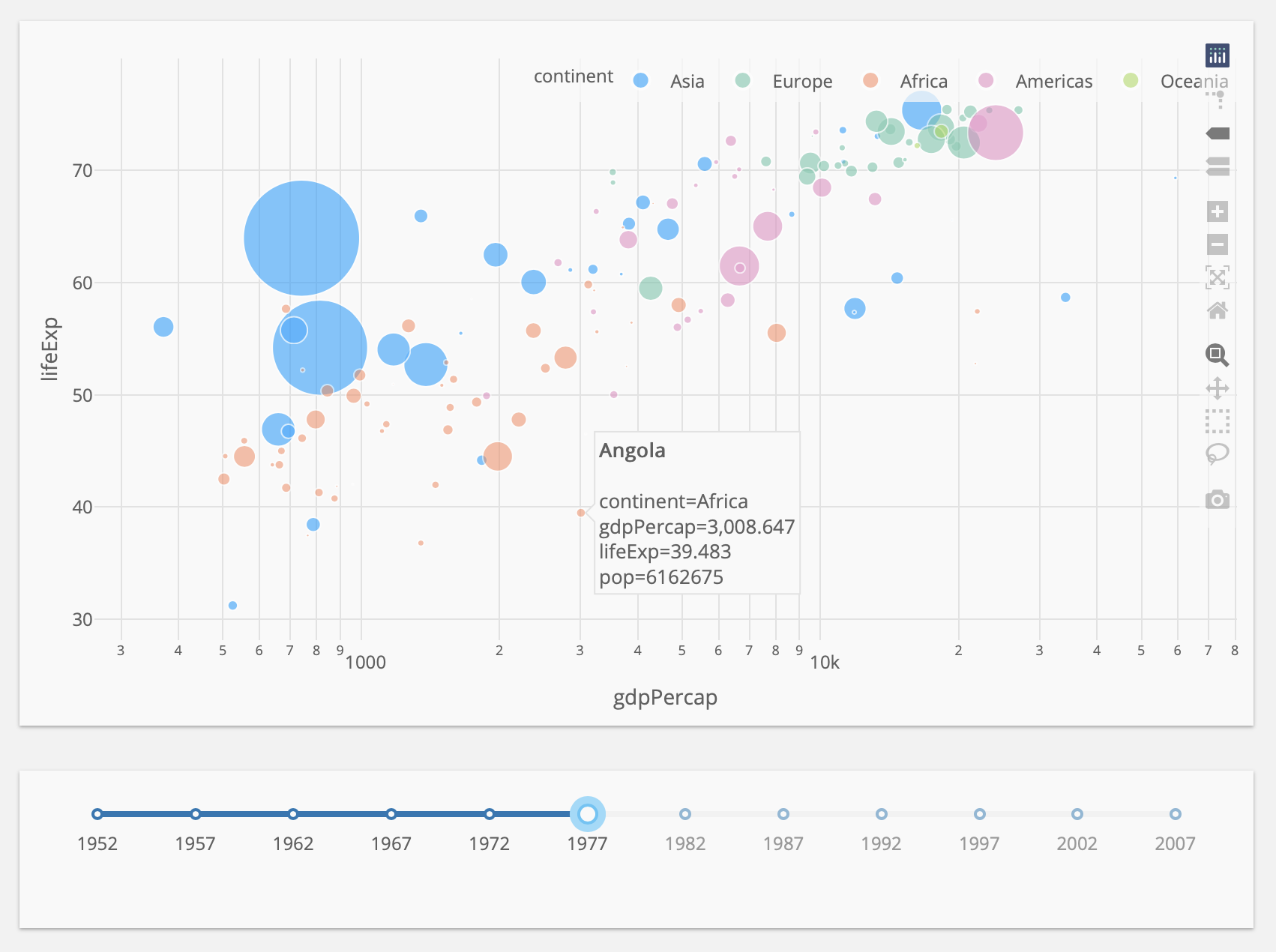Mars Theme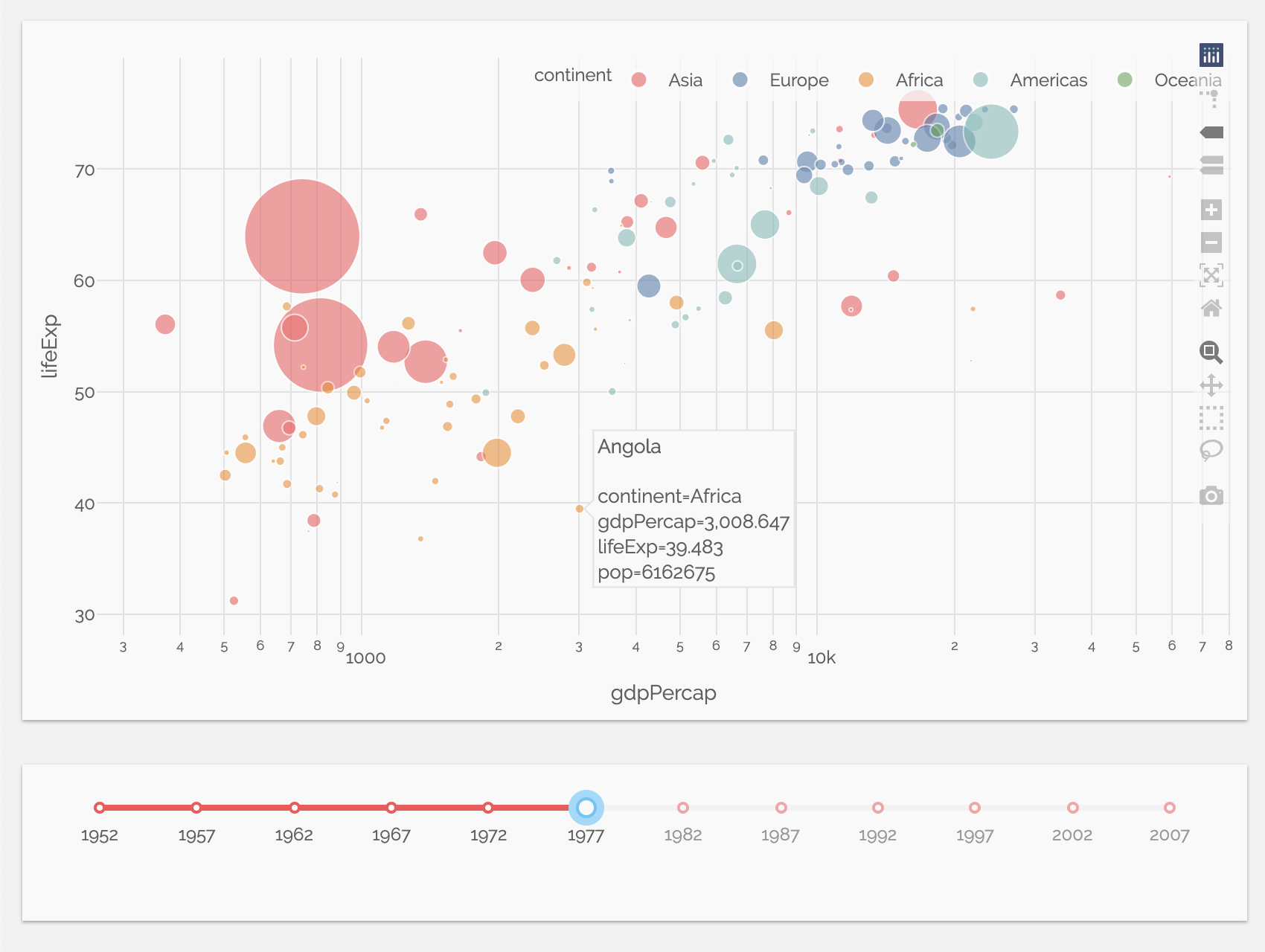Neptune Theme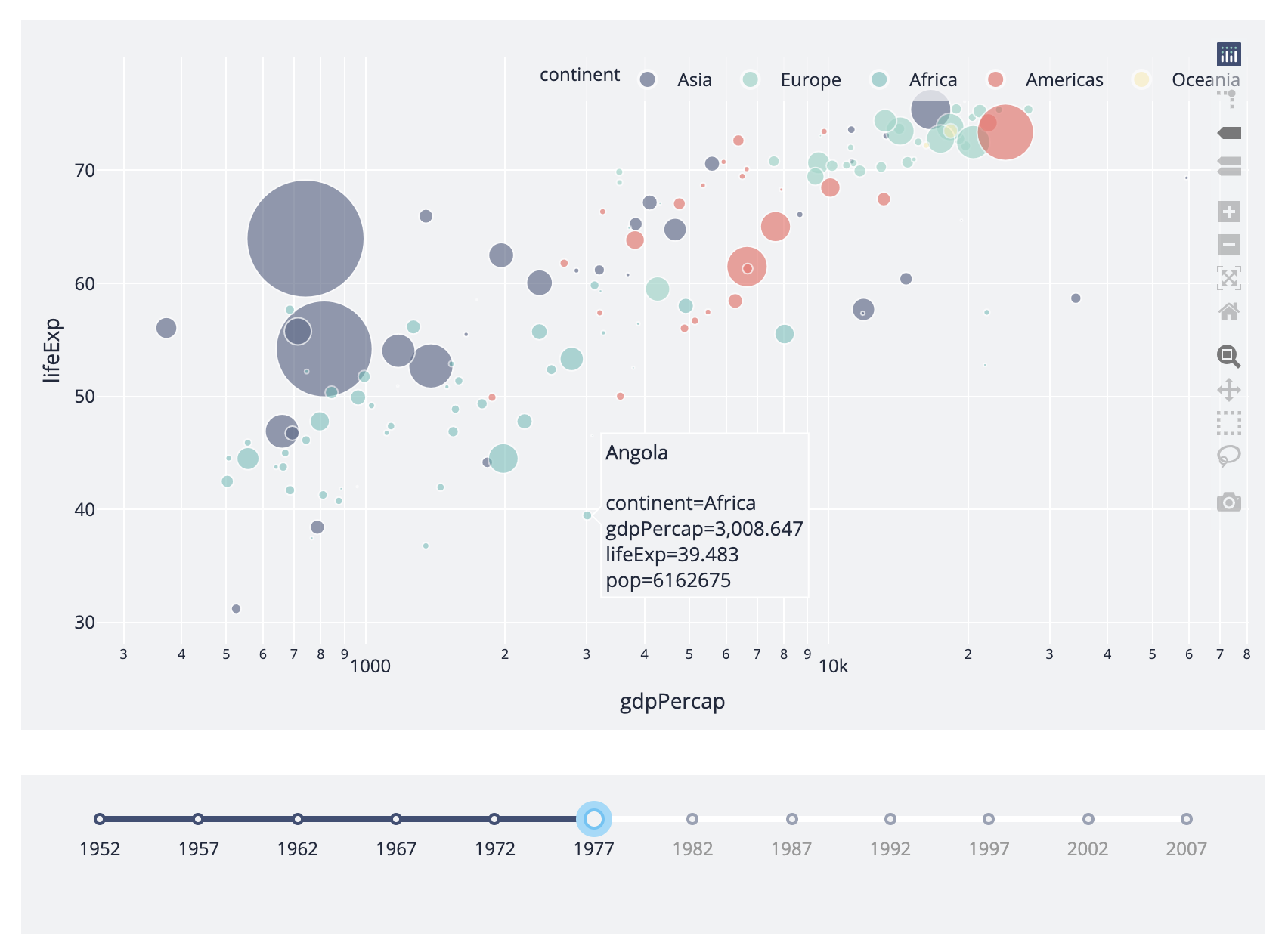Miller ThemeExtrasolar Theme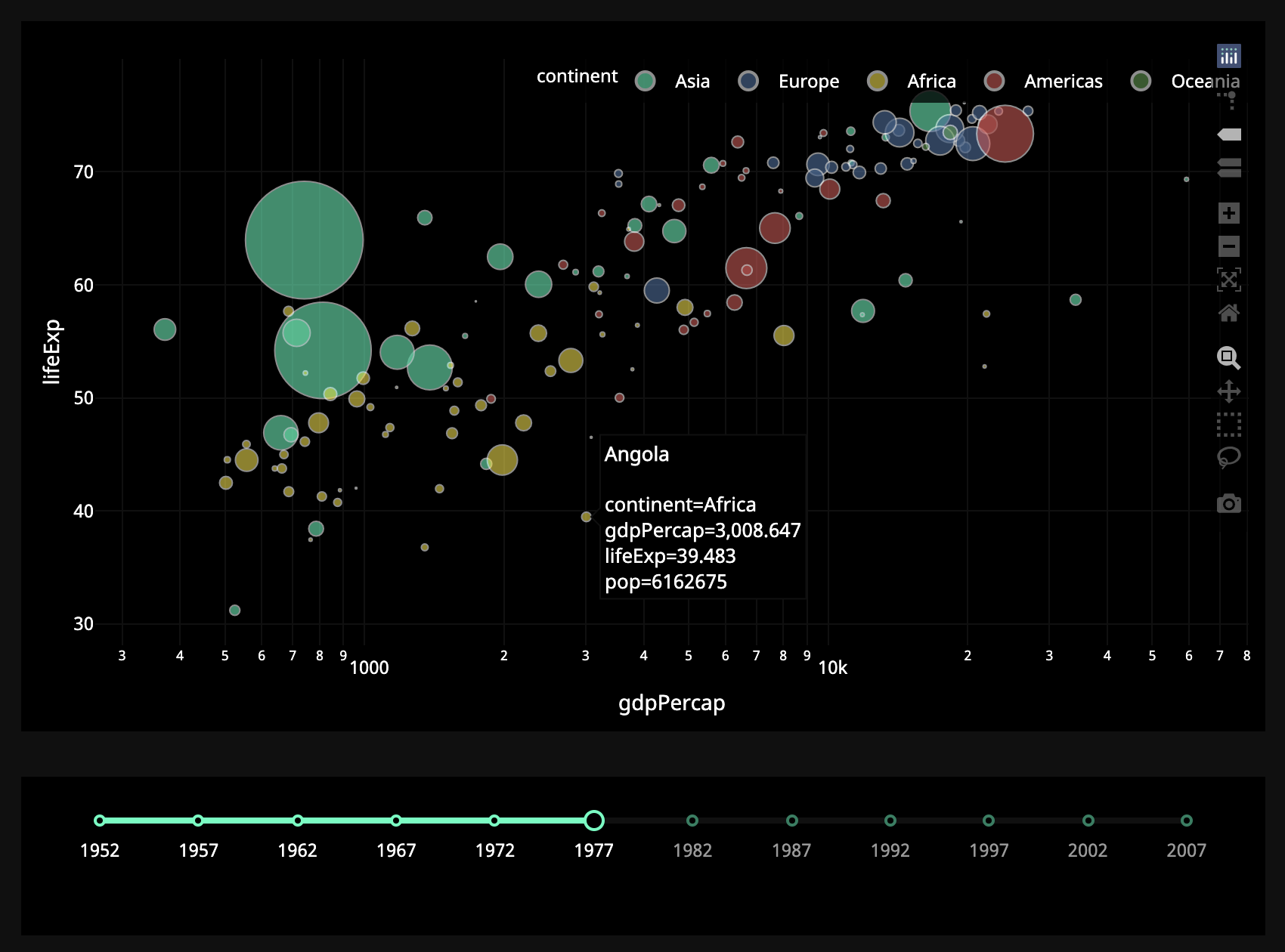Preset Themes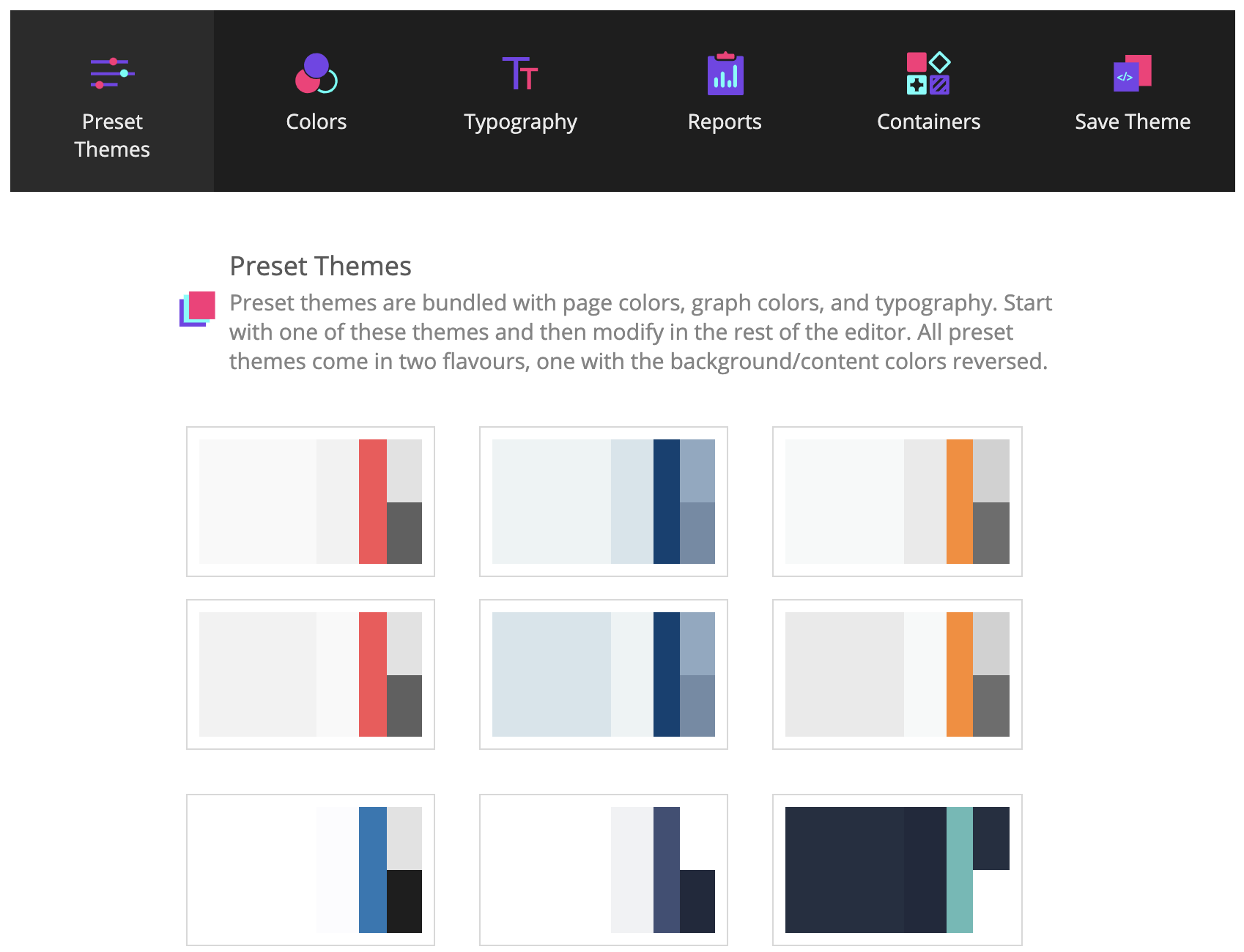In this example, the `"value"` property of the `dccSlider` is the
input of the app, and the output of the app is the `"figure"` property of the
`dccGraph`.
Whenever the `value` of the `dccSlider` changes, Dash calls the
callback function with the new value. The function filters the
dataframe with this new value, constructs a `figure` object,
and returns it to the Dash application.

There are a few nice patterns in this example:

1. We
load our dataframe at the start of the app:
`df <- read.csv('...')`.
This dataframe `df` is in the global state of the app and can be
the start of the app instead of inside the callback functions, we ensure
that this operation is only done once – when the app server starts. When a user
visits the app or interacts with the app, that data (`df`) is already in memory.
data) should be done in the global scope of the app instead of within
the callback functions.
3. The callback does not modify the original data, it only creates copies
of the dataframe by filtering .
This is important: your callbacks should never modify variables
outside of their scope
. If your callbacks modify global state, then one
user’s session might affect the next user’s session and when the app is
deployed on multiple processes or threads, those modifications will not
be shared across sessions.

#### Dash App With Multiple Inputs

In Dash, any “output” can have multiple “input” components.
Here’s a simple example that binds five inputs
(the `value` property of two `dccDropdown` components,
two `dccRadioItems` components, and one `dccSlider` component)
to one output component (the `figure` property of the `dccGraph` component).
Notice how `add_callback()` lists all five `input()`s after the `output()`. Also notice
that when there is more than one input, you need to place the inputs inside a `list`.

``````library(dash)
library(dashCoreComponents)
library(dplyr)

app <- dash_app()

file = 'https://gist.githubusercontent.com/chriddyp/cb5392c35661370d95f300086accea51/raw/8e0768211f6b747c0db42a9ce9a0937dafcbd8b2/indicators.csv',
stringsAsFactor = FALSE
)

available_indicators <- unique(df\$Indicator.Name)
years <- unique(df\$Year)
num_years <- length(years)

option_indicator <- lapply(
available_indicators,
function(available_indicator) {
list(label = available_indicator,
value = available_indicator)
}
)

app %>% set_layout(
div(
dccDropdown(
id = 'xaxis-column',
options = option_indicator,
value = 'Fertility rate, total (births per woman)'
),
id = 'xaxis-type',
options = list(list(label = 'Linear', value = 'linear'),
list(label = 'Log', value = 'log')),
value = 'linear',
labelStyle = list(display = 'inline-block')
),
style = list(width = '48%', display = 'inline-block')
),
div(
dccDropdown(
id = 'yaxis-column',
options = option_indicator,
value = 'Life expectancy at birth, total (years)'
),
id = 'yaxis-type',
options = list(list(label = 'Linear', value = 'linear'),
list(label = 'Log', value = 'log')),
value = 'linear',
labelStyle = list(display = 'inline-block')
),
style = list(width = '48%', float = 'right', display = 'inline-block')
),
dccGraph(id = 'indicator-graphic'),
dccSlider(
id = 'year--slider',
min = 0,
max = num_years - 1,
marks = years,
value = num_years - 1
)
)

output('indicator-graphic', 'figure'),
list(
input('xaxis-column', 'value'),
input('yaxis-column', 'value'),
input('xaxis-type', 'value'),
input('yaxis-type', 'value'),
input('year--slider', 'value')
),
function(xaxis_column_name, yaxis_column_name, xaxis_type, yaxis_type, year_value) {
data_by_indicator <- df %>%
dplyr::filter(Year == years[year_value + 1],
Indicator.Name %in% c(xaxis_column_name,
yaxis_column_name))  %>%
droplevels() %>%
split(., .\$Indicator.Name)

filtered_df <- merge(data_by_indicator[], data_by_indicator[], by = "Country.Name") %>%
dplyr::transmute(x = Value.x, y = Value.y, text = Country.Name) %>%
na.omit() %>%
as.list()

inputData <- list(
c(
filtered_df,
list(
opacity = 0.7,
mode = 'markers',
marker = list(
size = 15,
line = list(width = 0.5, color = 'white')
)
)
)
)

list(
data = inputData,
layout = list(
xaxis = list('title' = xaxis_column_name, 'type' = xaxis_type),
yaxis = list('title' = yaxis_column_name, 'type' = yaxis_type),
margin = list('l' = 40, 'b' = 40, 't' = 10, 'r' = 10),
legend = list('x' = 0, 'y' = 1),
hovermode = 'closest'
)
)
}
)

app %>% run_app()
``````

Theming with Dash Enterprise Design Kit

Default Theme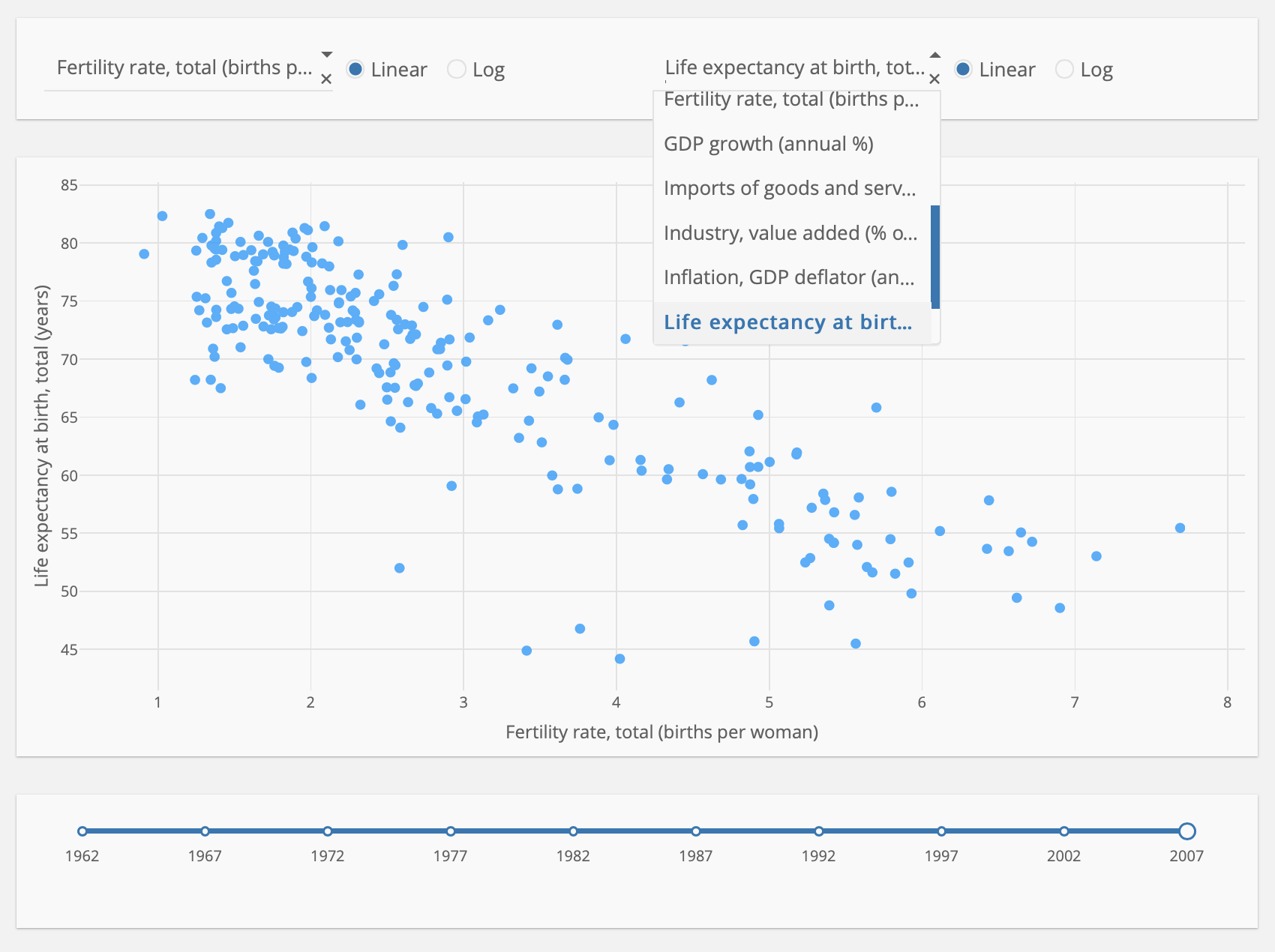Mars Theme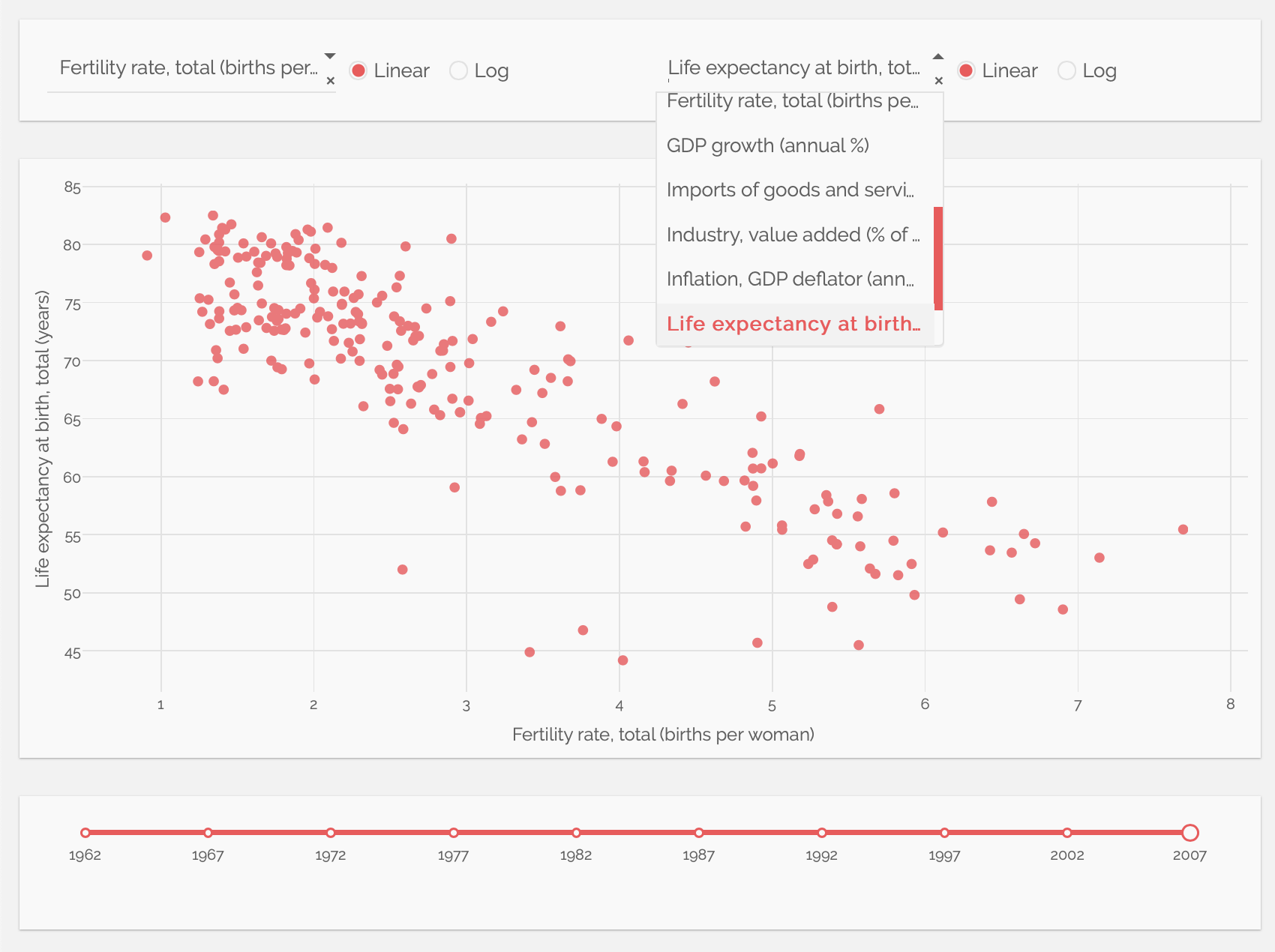Neptune Theme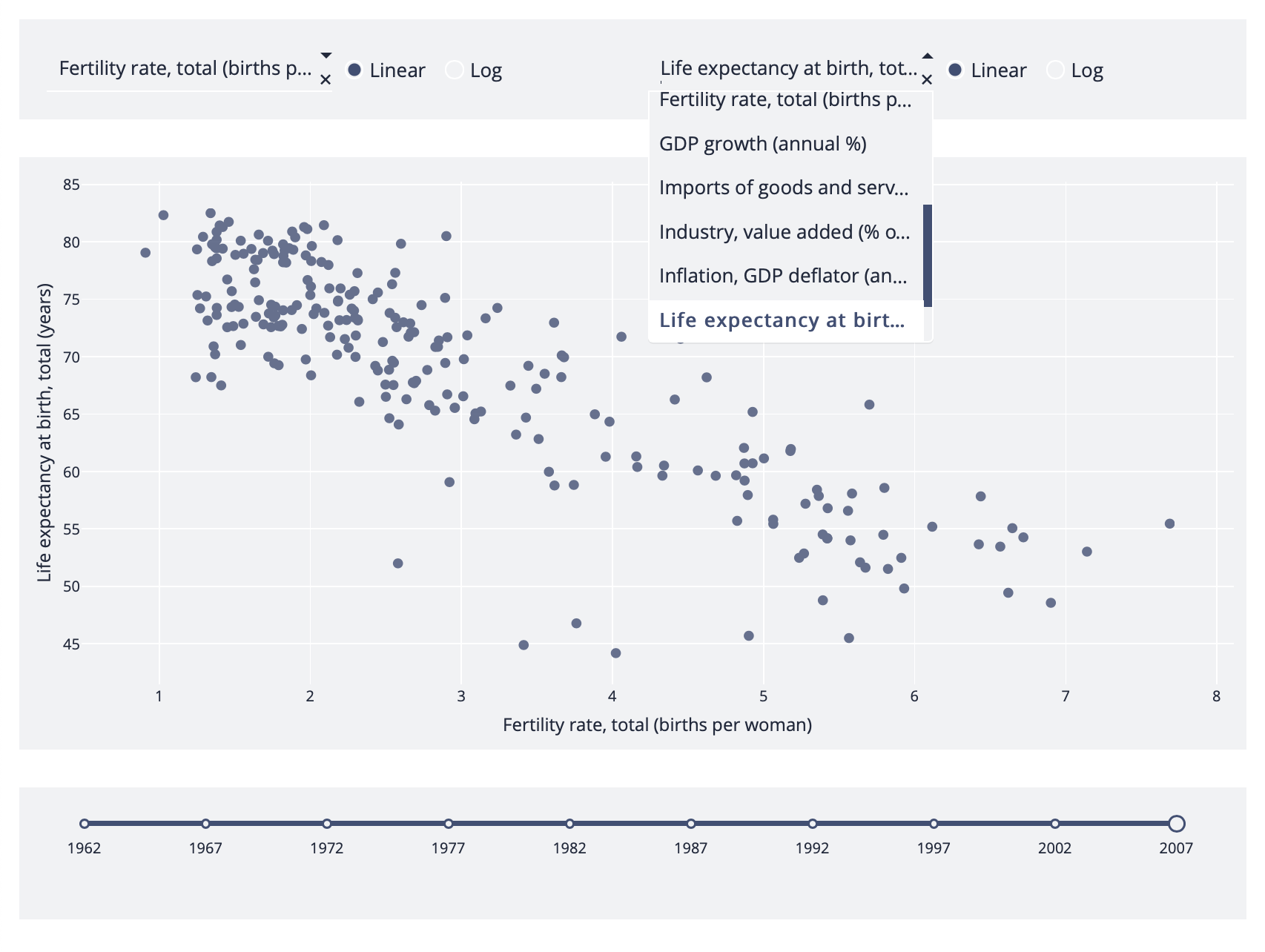Miller Theme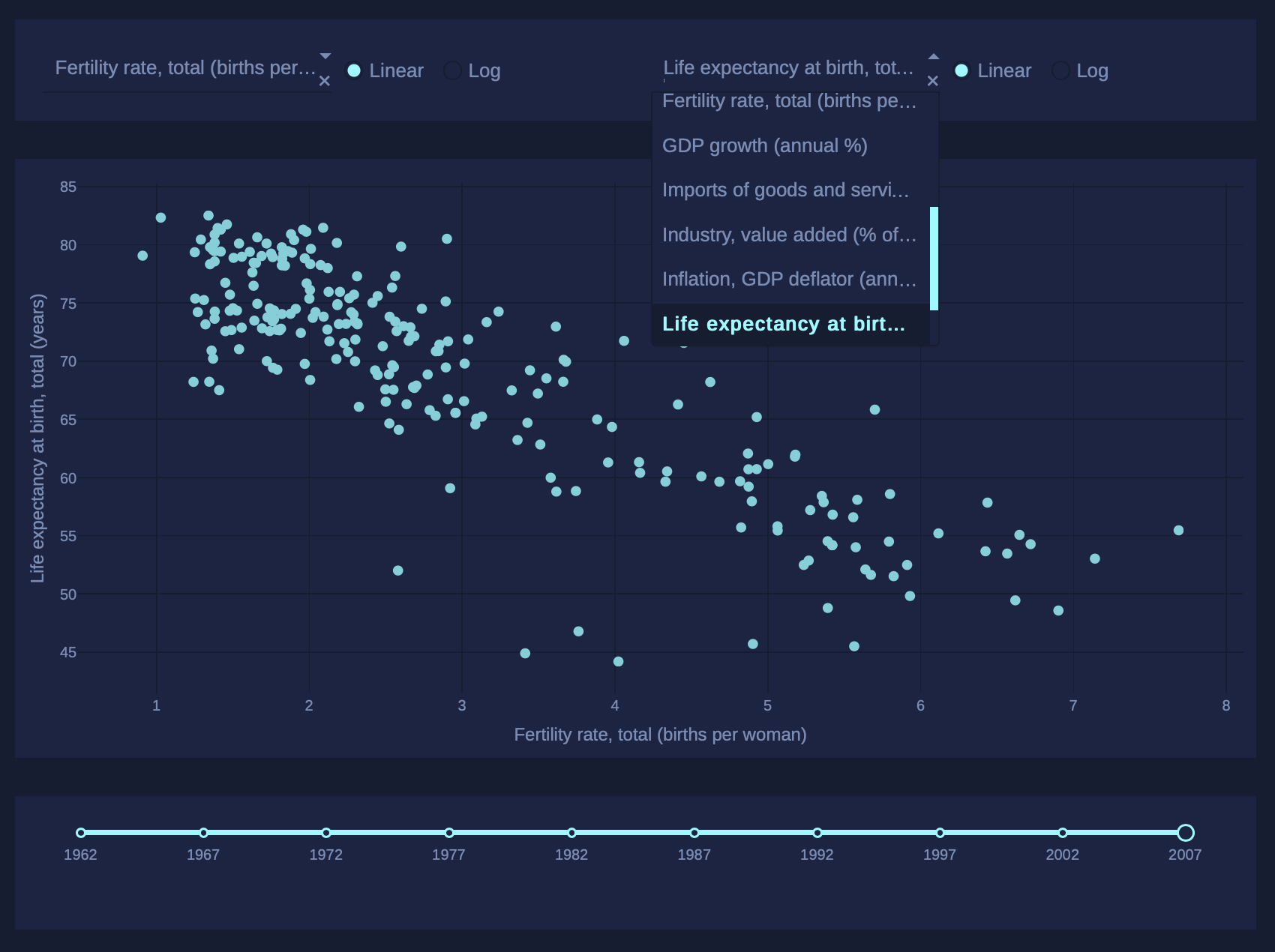Extrasolar Theme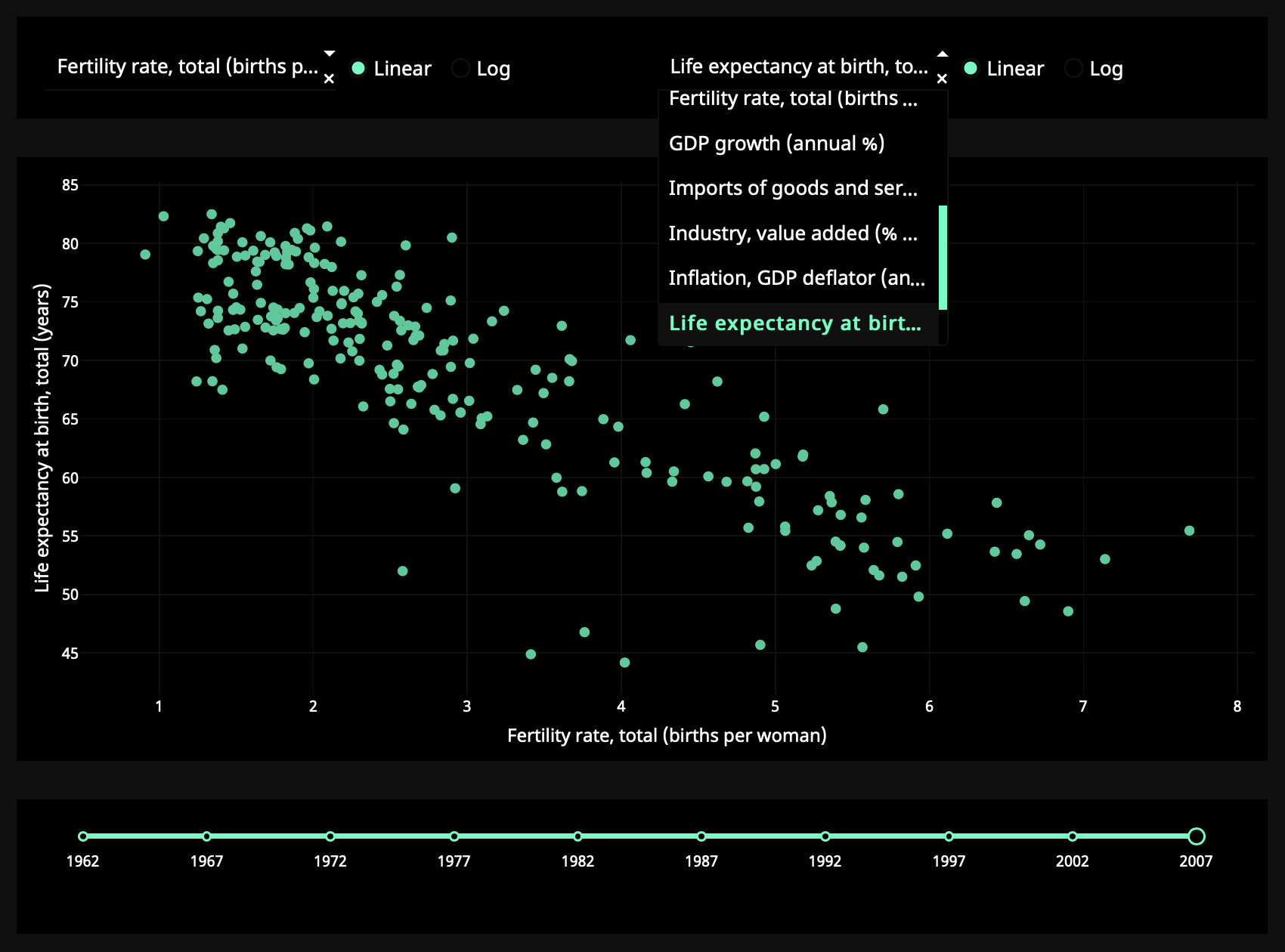Design Kit Theme EditorIn this example, the callback executes whenever the `value` property of any of the
`dccDropdown`, `dccSlider`,
or `dccRadioItems` components change.

The input arguments of the callback are the current
value of each of the “input” properties, in the order that they were
specified.

Even though only a single input changes at a time (i.e. a user can only change
the value of a single Dropdown in a given moment), Dash collects the
current state of all the specified input properties and passes them
into the callback function. These callback functions are always guaranteed
to receive the updated state of the app.

Let’s extend our example to include multiple outputs.

#### Dash App With Multiple Outputs

So far all the callbacks we’ve written only update a single output property.
We can also update several outputs at once: list all the properties you want to update
inside a `list` in `add_callback()`,
and return that many items from the callback. This is particularly useful if
two outputs depend on the same computationally intensive intermediate result,
such as a slow database query.

``````library(dash)
library(dashCoreComponents)

app <- dash_app()

app %>% set_layout(
dccInput(
id = 'num-multi',
type = 'number',
value = 1
),
html\$table(
html\$tr(html\$td('x', html\$sup(2)), html\$td(id='square')),
html\$tr(html\$td('x', html\$sup(3)), html\$td(id='cube')),
html\$tr(html\$td('2', html\$sup('x')), html\$td(id='twos')),
html\$tr(html\$td('3', html\$sup('x')), html\$td(id='threes')),
html\$tr(html\$td('x', html\$sup('x')), html\$td(id='xx'))
)
)

list(
output('square', 'children'),
output('cube', 'children'),
output('twos', 'children'),
output('threes', 'children'),
output('xx', 'children')
),
input('num-multi', 'value'),
function(x) {
list(x**2, x**3, 2**x, 3**x, x**x)
}
)

app %>% run_app()
``````
 x 2 x 3 2 x 3 x x x

Note that you can update multiple properties of the same component,
or you can even update multiple different components. Also note that when a callback has multiple outputs,
you need to return all the different outputs in a `list`.

A word of caution: it’s not always a good idea to combine outputs, even if
you can:

• If the outputs depend on some, but not all, of the same inputs, then keeping
them separate can avoid unnecessary updates.
• If the outputs have the same inputs but they perform very different computations with these
inputs, keeping the callbacks separate can allow them to run in parallel.

#### Dash App With Chained Callbacks

You can also chain outputs and inputs together: the output of one callback
function could be the input of another callback function.

This pattern can be used to create dynamic UIs where, for example, one input component
updates the available options of another input component.
Here’s a simple example.

``````library(dash)
library(dashCoreComponents)

app <- dash_app()

all_options <- list(
'America' = list('New York City', 'San Francisco', 'Cincinnati'),
)

app %>% set_layout(
options = list(list(label = 'America', value = 'America'),
value = 'America'
),
html\$hr(),
html\$hr(),
div(id = 'display-selected-values')
)

function(selected_country) {
data_selected <- all_options[[selected_country]]
lapply(data_selected,
function(dat) {
list('label' = dat,
'value' = dat)
})
})

function(option) NULL
)

output('display-selected-values', 'children'),
list(
),
function(selected_country, selected_city) {
sprintf("\"%s\ is a city in \"%s\"", selected_city, selected_country)
})

app %>% run_app()
``````

The first callback updates the available options in the second
`dccRadioItems` component based off of the selected value in the
first `dccRadioItems` component.

The second callback sets an initial value when the `options` property
changes: it sets it to the first value in that `options` list.

The final callback displays the selected `value` of each component.
If you change the `value` of the countries `dccRadioItems`
component, Dash will wait until the `value` of the cities component is updated
before calling the final callback. This prevents your callbacks from being
called with inconsistent state like with `"America"` and `"Montréal"`.

#### Dash App With State

In some cases, you might have a “form”-like pattern in your
application. In such a situation, you may want to read the value
of an input component, but only when the user is finished
entering all of their information in the form rather than immediately after
it changes.

Attaching a callback to the input values directly can look like this:

``````library(dash)
library(dashCoreComponents)

app <- dash_app()

app %>% set_layout(
dccInput(id = 'input-1', type = 'text', value = 'Montreal'),
dccInput(id = 'input-2', type = 'text', value = 'Canada'),
div(id = 'output_keywords')
)

output('output_keywords', 'children'),
list(
input('input-1', 'value'),
input('input-2', 'value')
),
function(input1, input2) {
sprintf("Input 1 is \"%s\" and Input 2 is \"%s\"", input1, input2)
}
)

app %>% run_app()
``````

In this example, the callback function is fired whenever any of the
attributes described by the inputs change.
Try it for yourself by entering data in the inputs above.

`"state"` allows you to pass along extra values without
firing the callbacks. Here’s the same example as above but with the two
`dccInput` components as states
and a new button component as an input.

``````library(dash)
library(dashCoreComponents)

app <- dash_app()

app %>% set_layout(
dccInput(id = 'input-1-state', type = 'text', value = 'Montreal'),
dccInput(id = 'input-2-state', type = 'text', value = 'Canada'),
button(id = 'submit-button', n_clicks = 0, 'Submit'),
div(id = 'output-state')
)

output('output-state', 'children'),
list(
input('submit-button', 'n_clicks'),
state('input-1-state', 'value'),
state('input-2-state', 'value')
),
function(n_clicks, input1, input2) {
sprintf("The Button has been pressed \"%s\" times, Input 1 is \"%s\", and Input 2 is \"%s\"", n_clicks, input1, input2)
}
)

app %>% run_app()
``````

In this example, changing text in the `dccInput` boxes won’t fire
the callback, but clicking on the button will. The current values of the
`dccInput` values are still passed into the callback even though
they don’t trigger the callback function itself.

Note that we’re triggering the callback by listening to the `n_clicks` property
of the `button` component. `n_clicks` is a property that gets
incremented every time the component has been clicked on.
It’s available in every pure HTML component in
Dash package, but most useful with buttons.

#### Summary

We’ve covered the fundamentals of callbacks in Dash.
Dash apps are built off of a set
of simple but powerful principles: UIs that are customizable
through reactive callbacks.
Every attribute/property of a component can be modified
as the output of a callback, while a subset of the attributes (such as the `value`
property of `dccDropdown` component)
are editable by the user through interacting with the page.

The next part of the Dash tutorial covers interactive graphing. Dash Tutorial Part 4: Interactive Graphing